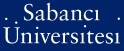# Introduction to stochastic programming and its applications to finance

Şimşek, Koray Deniz (2008) Introduction to stochastic programming and its applications to finance. In: Fabozzi, Frank J., (ed.) Handbook of Finance: Valuation, Financial Modeling, and Quantitative Tools, Volume III. Wiley, Hoboken, NJ, USA, pp. 775-783. ISBN 978-0-470-04256-4

This is the latest version of this item.PDF (Book Chapter - Published) - Repository staff only - Requires a PDF viewer such as GSview, Xpdf or Adobe Acrobat Reader544Kb

## Abstract

Mathematical programming is one of a number of operations research techniques that employs mathematical optimization models to assist in decision making. Mathematical programming includes linear programming, integer programming, mixed-integer programming, nonlinear programming, stochastic programming, and goal programming. Mathematical programming models allow the decision maker to identify the “best” solution. This is in contrast to other mathematical tools that are in the arsenal of decision makers such as statistical models (which tell the decision maker what occurred in the past), forecasting models (which tell the decision maker what might happen in the future), and simulation models (which tell the decision maker what will happens under different conditions). The mean-variance model for portfolio selection as formulated by Markowitz is an example of an application of one type of mathematical programming (quadratic programming). However, in formulating optimization models in many applications in finance, the mathematical programming model employed needs to take into consideration the uncertainty about the model’s parameters and the multi-period nature of the problem faced. To deal with these situations, the technique of stochastic programming is employed.

Item Type: Book Section / Chapter H Social Sciences > HG Finance 10249 Koray Deniz Şimşek 09 Nov 2008 22:08 13 Nov 2008 09:16

### Available Versions of this Item

Repository Staff Only: item control page